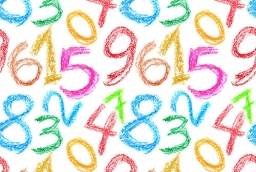# Difference 5847

I reduced the unknown number by five and multiplied the result difference by three. Finally, I increase the resulting product by six and get the least common multiple of the numbers 3 and 8. Calculate the unknown number.

n =  11

### Step-by-step explanation:

(n-5)·3+6 = 3·8

3n = 33

n = 33/3 = 11

n = 11

Our simple equation calculator calculates it.Did you find an error or inaccuracy? Feel free to write us. Thank you!

Tips for related online calculators
Do you want to calculate the least common multiple of two or more numbers?
Do you have a linear equation or system of equations and looking for its solution? Or do you have a quadratic equation?